﻿ Set Of Geometric Shapes, Triangles, Line Design, Stock Vector - Illustration of design, line: 50392948

Set of geometric shapes, triangles, line design, .

Set of geometric shapes, triangles, line design

Designers Also Selected These Stock IllustrationsDinosaur silhouettes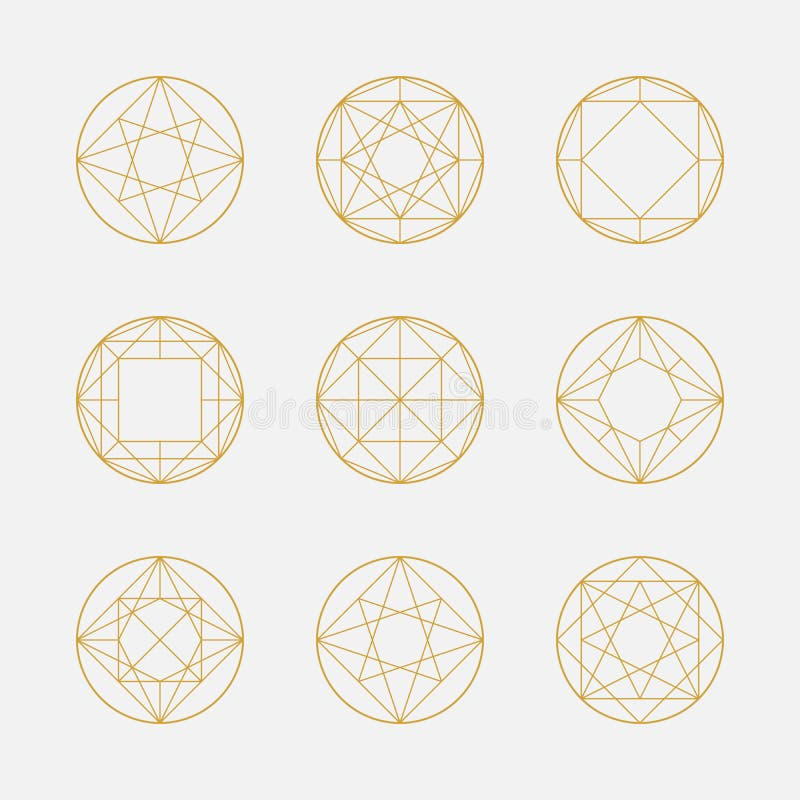Set of geometric shapes, squares and circles, line design,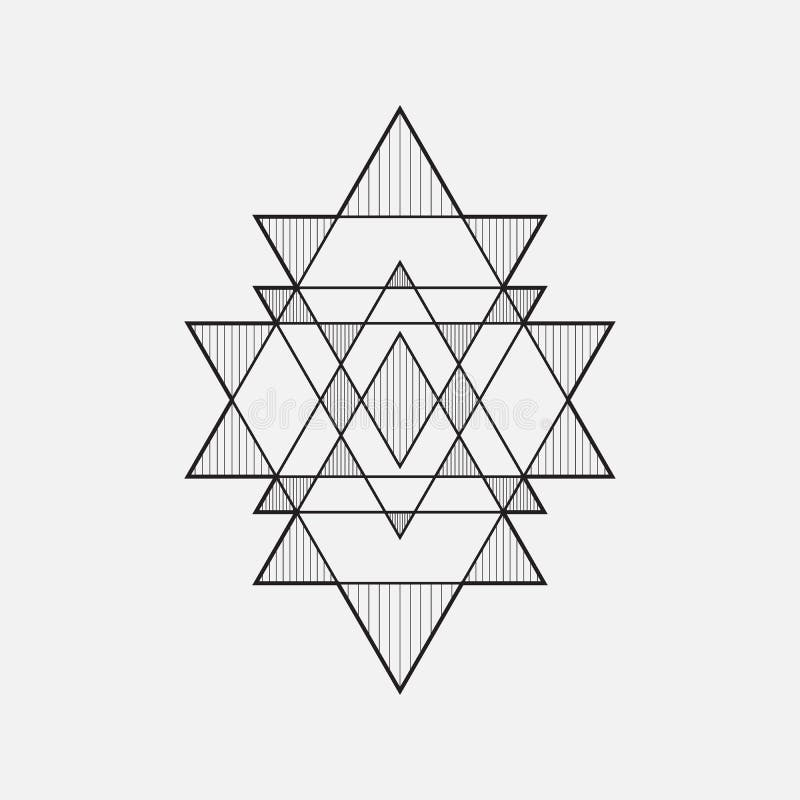Geometric shapes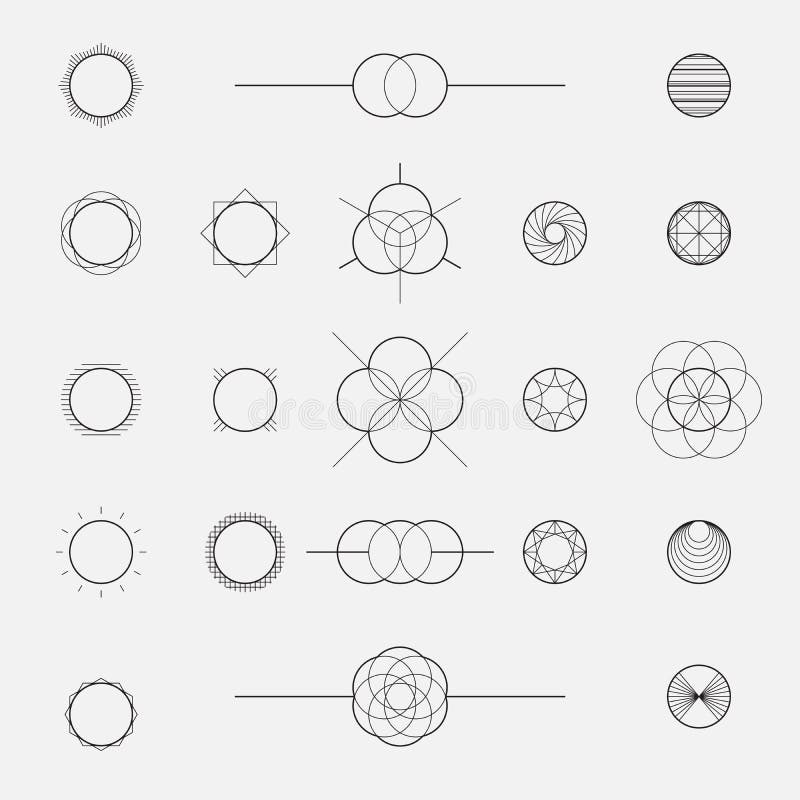Set of geometric shapes, circles, line design, vector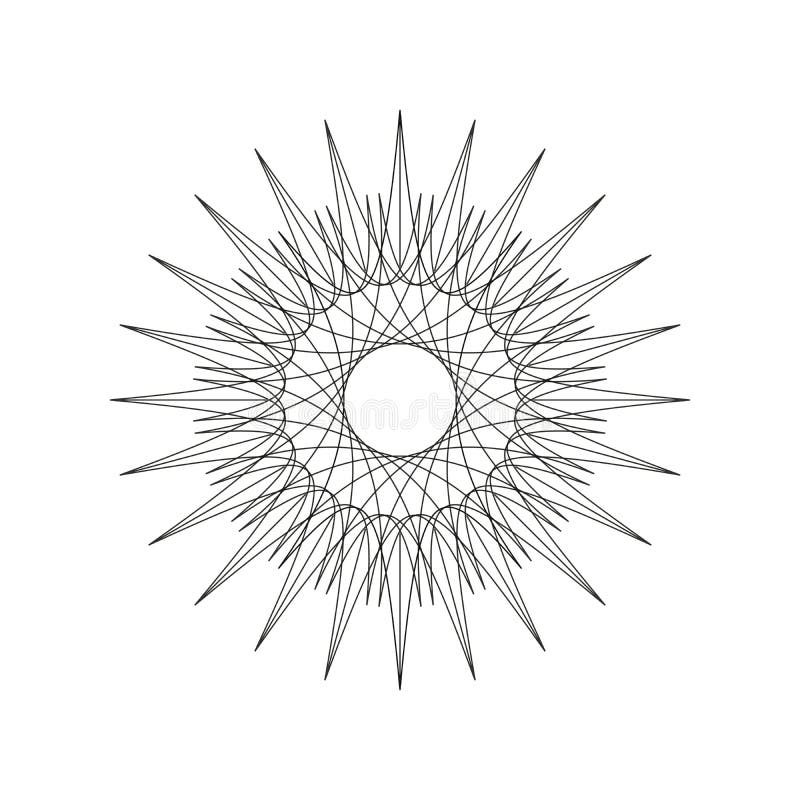Geometric decoration shape, ornament mandala line design, illustration eps 10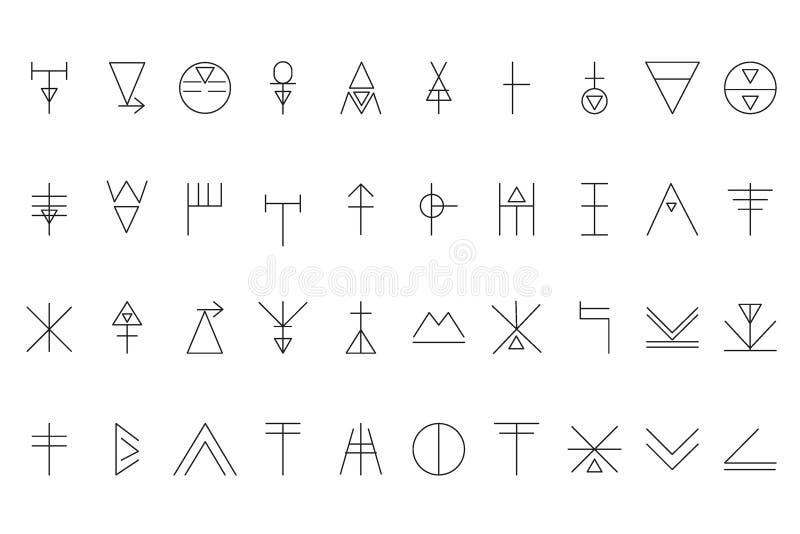Set of geometric shapes5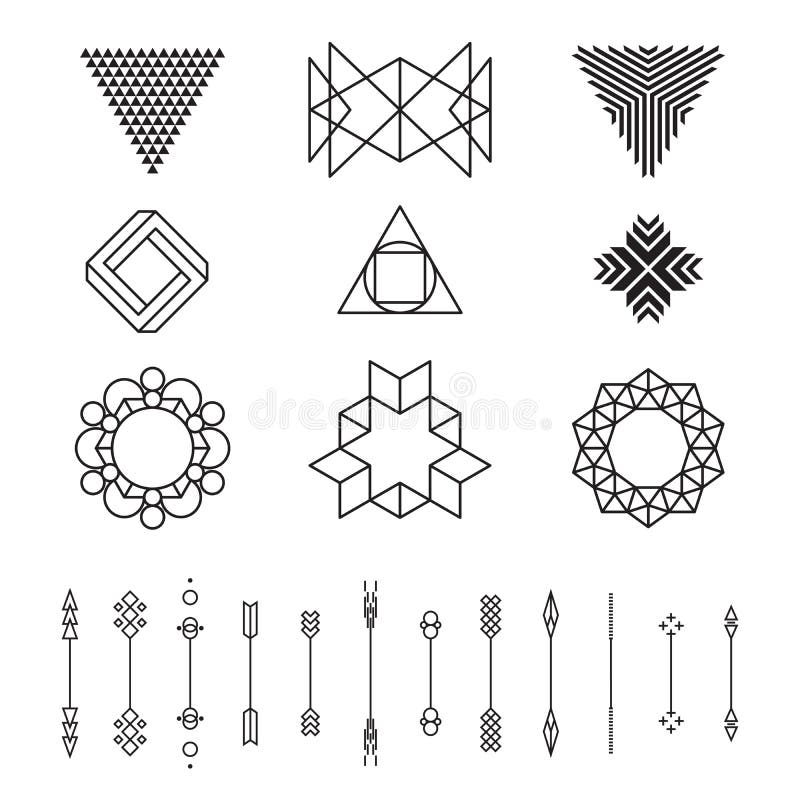Set of geometric shapes, vector illustration, isolated, line design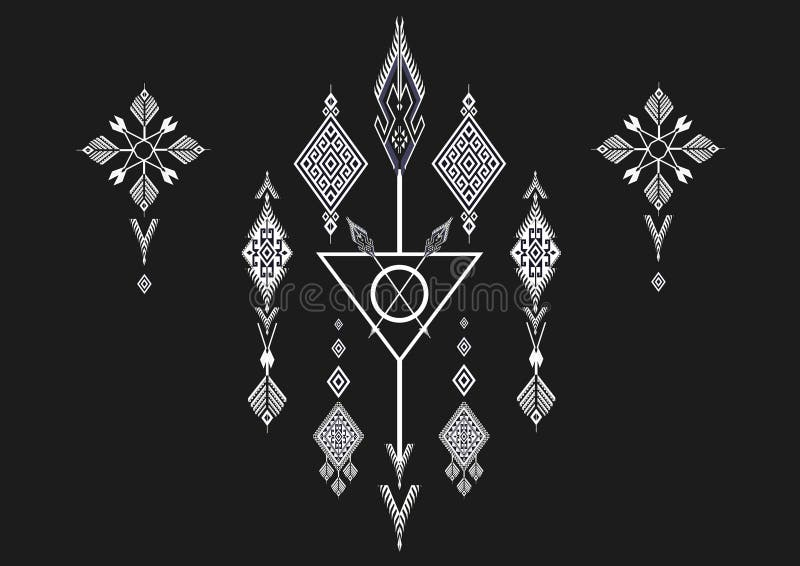Geometric Ethnic pattern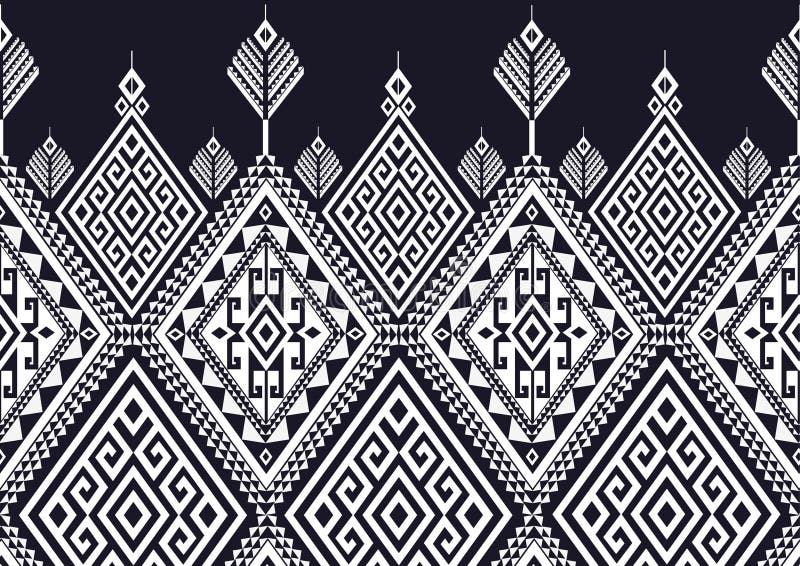Geometric Ethnic pattern
More similar stock illustrations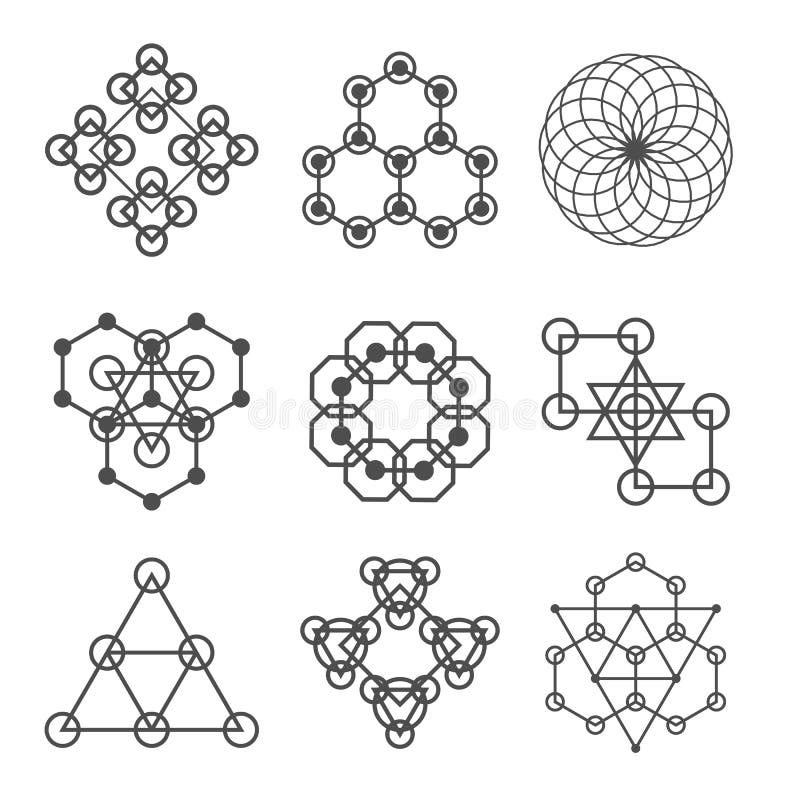Set of line abstract geometric logotypes. Set of abstract geometric shapes, triangles, line design, logos.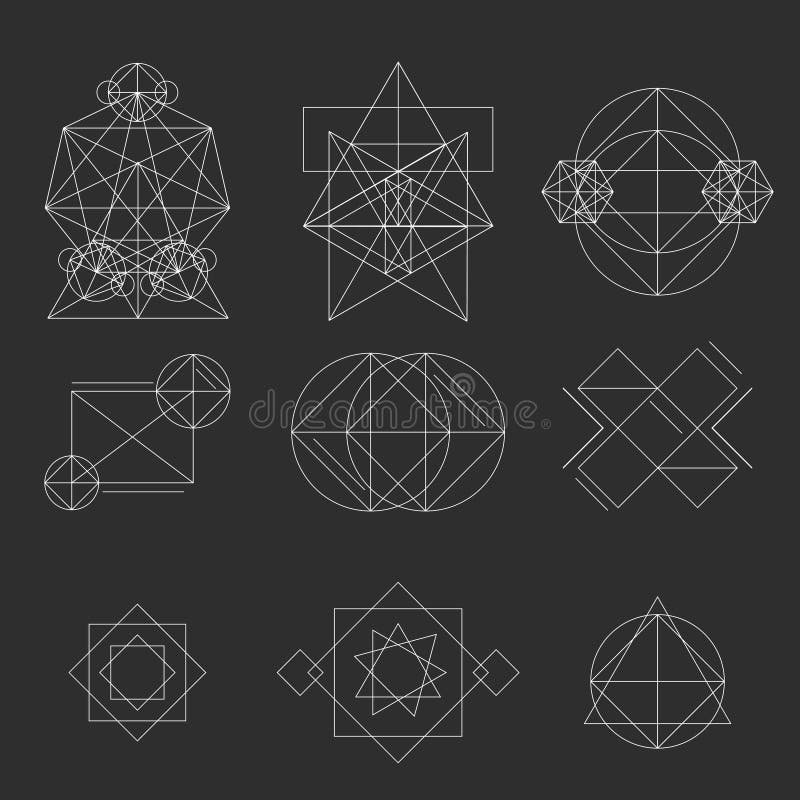Set geometric signs, labels, and frames. Triangles. Line design elements, illustration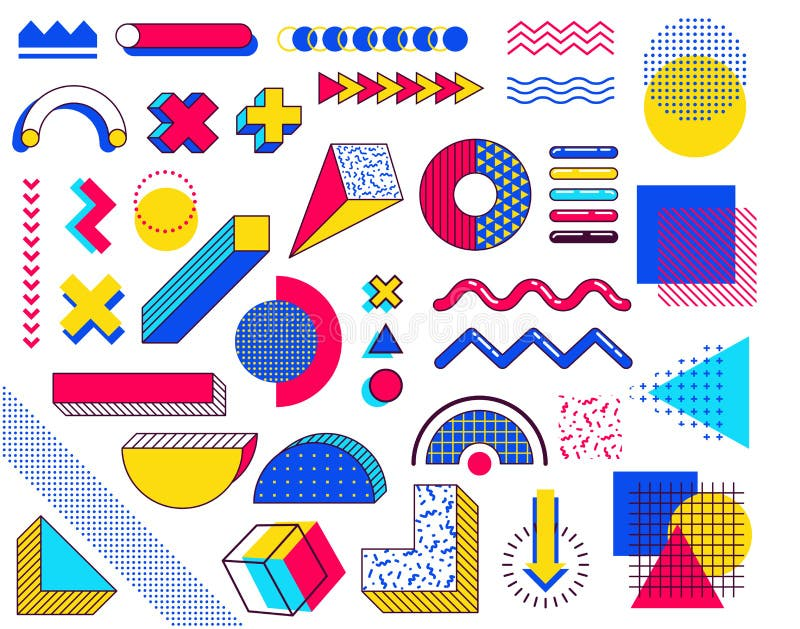Set of memphis design elements. Abstract 90s trends elements with multicolored simple geometric shapes. Shapes with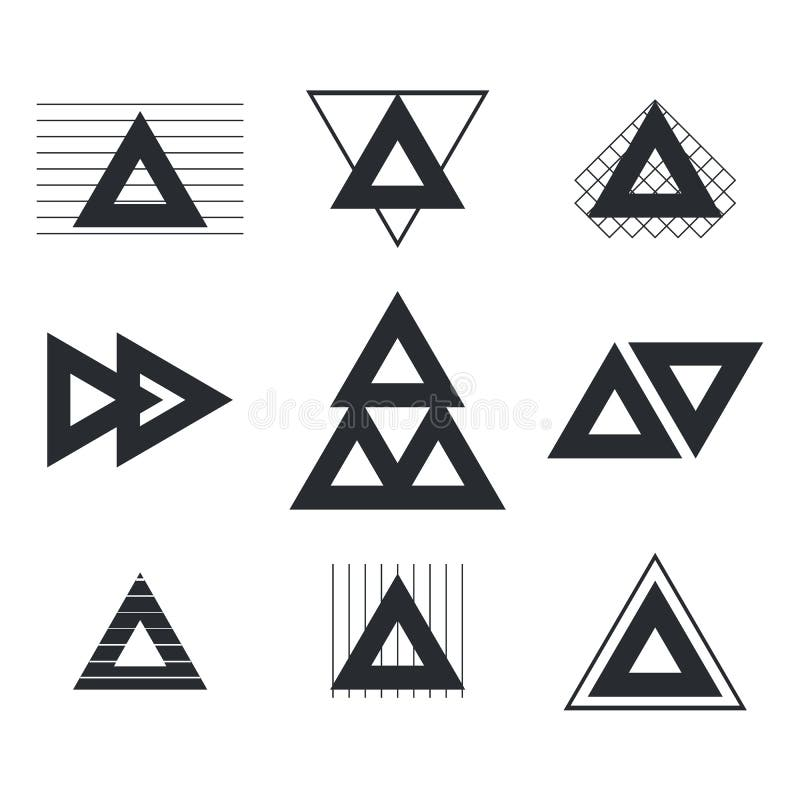Set of geometric shapes triangles, lines for your design. Trendy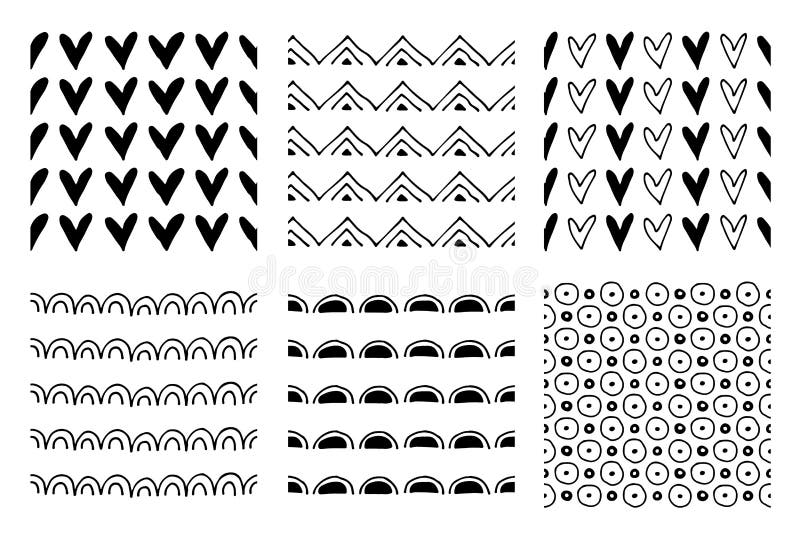Set of seamless vector patterns. Black and white geometrical endless backgrounds with hand drawn geometric shapes, triangles, circ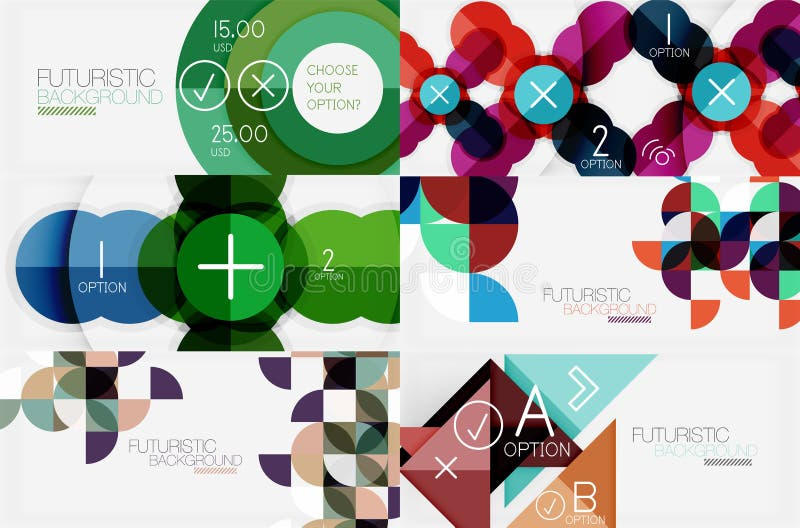Set of minimalistic geometric banners with triangles and circles and other shapes. Web design or business slogan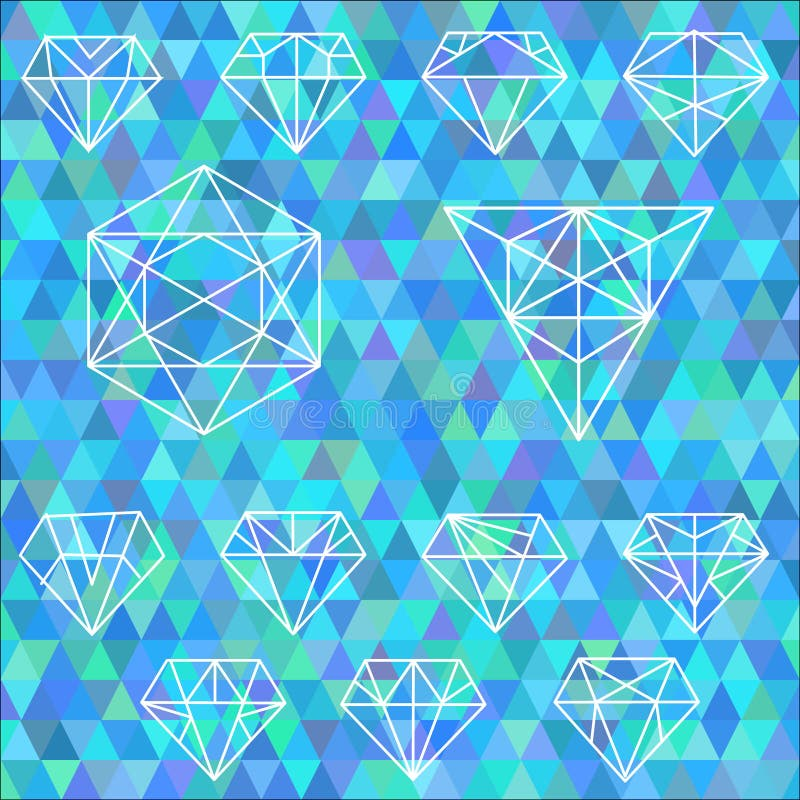The set of linear geometric shapes. Hexagons, triangles, crystal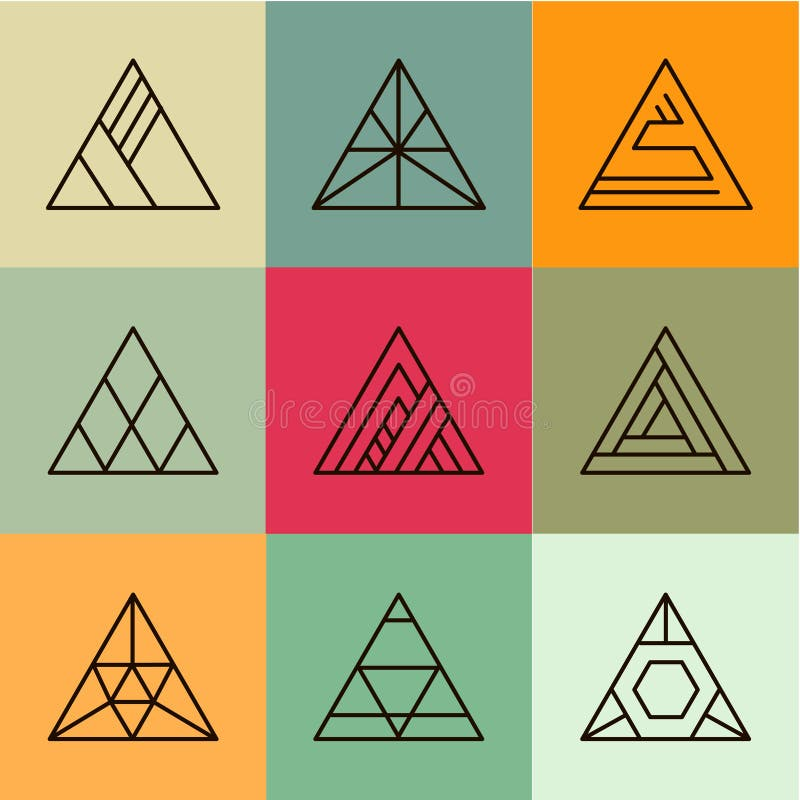Set of geometric shapes, triangles. Trendy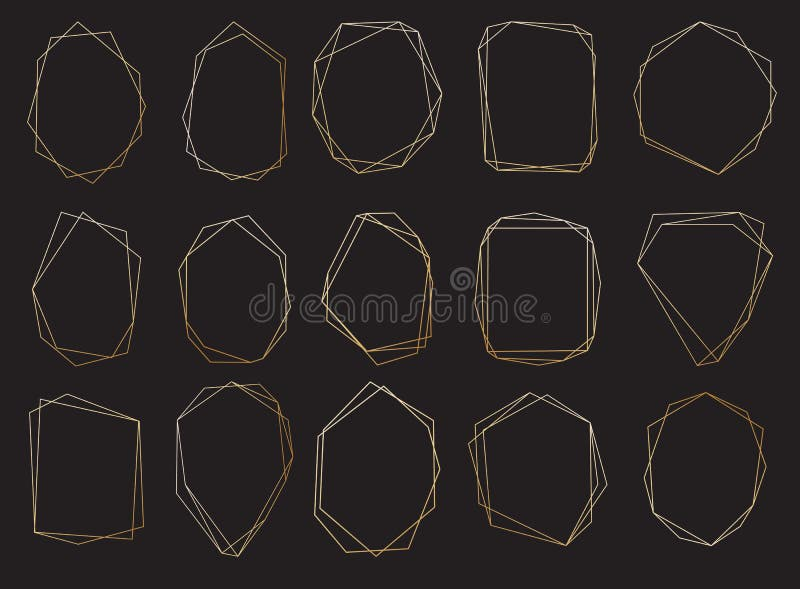Polygonal frames set. Gold triangles, geometric shapes.
More stock photos from Tomas Kovalcik's portfolio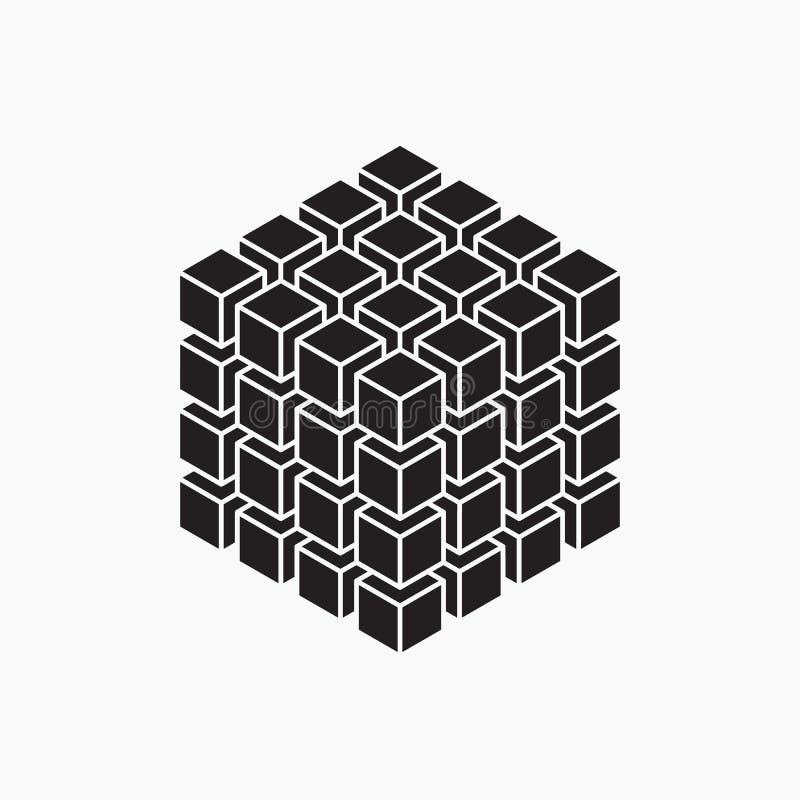Cube, geometric element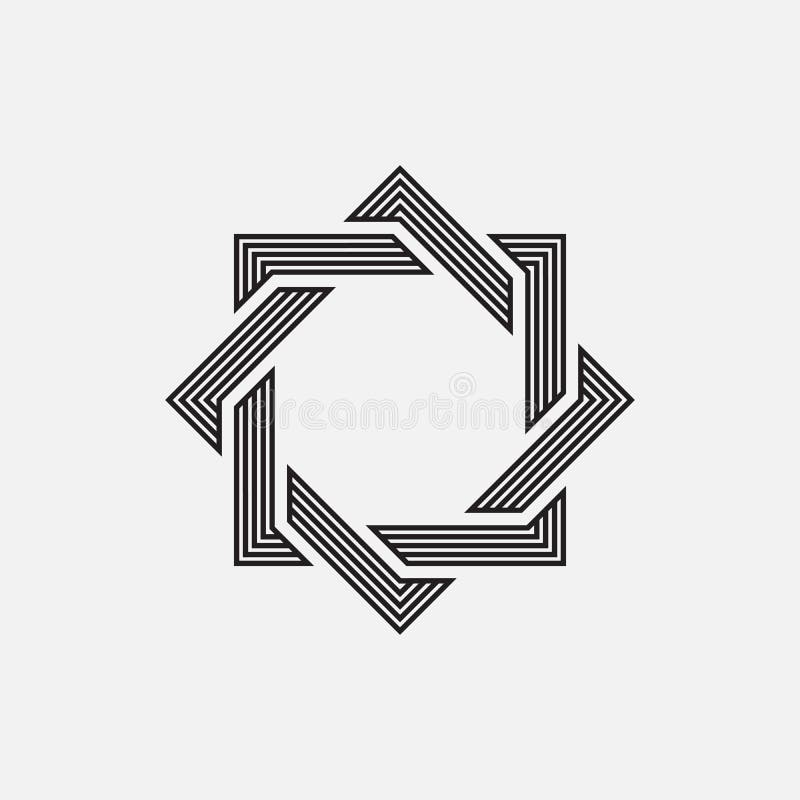Intertwined geometric shapesGeometric elements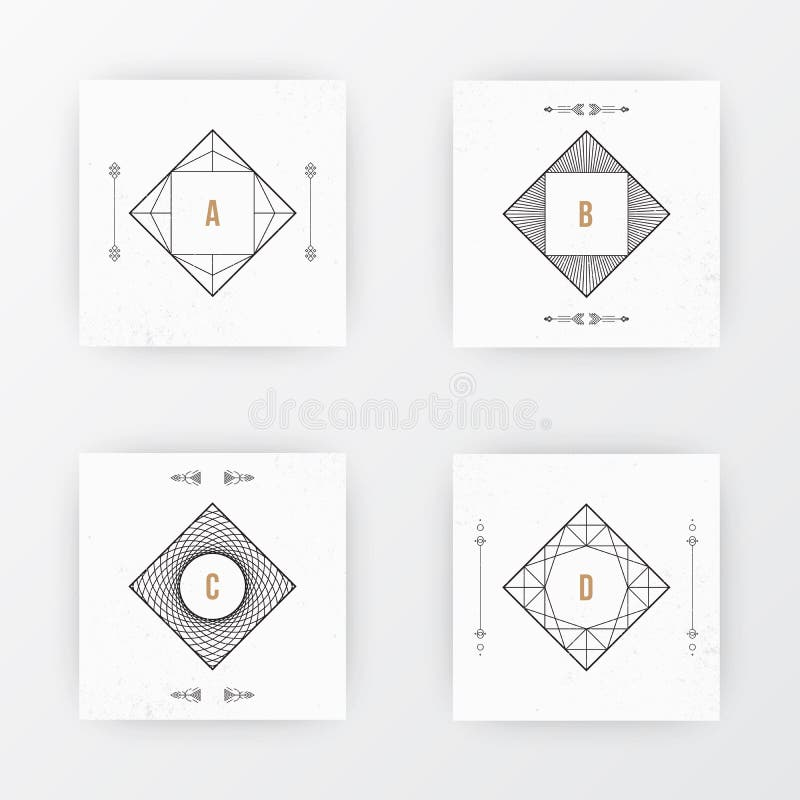Square brochure template design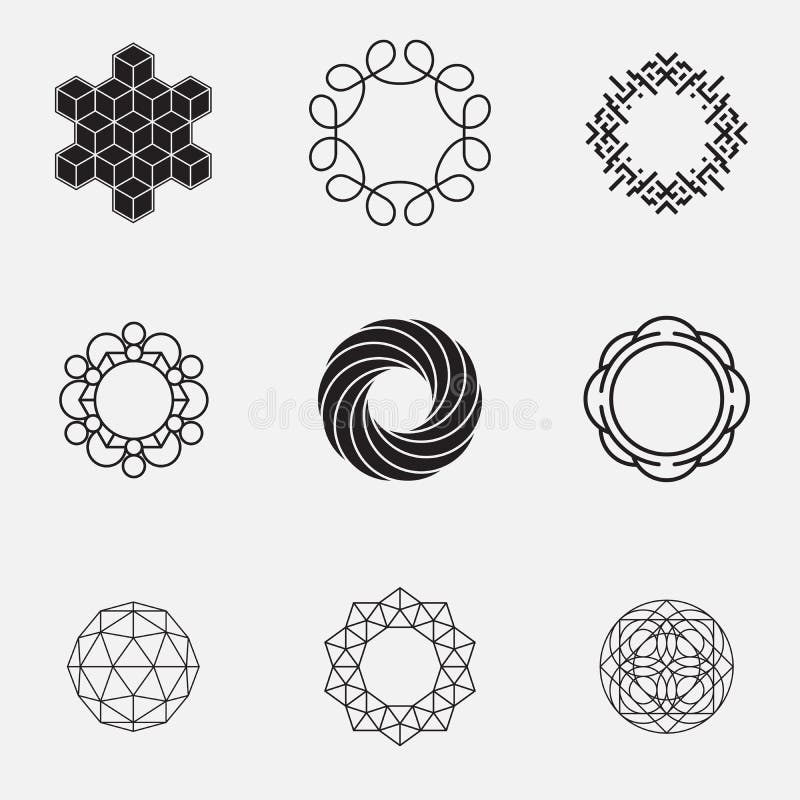Set of geometric shapes, circles,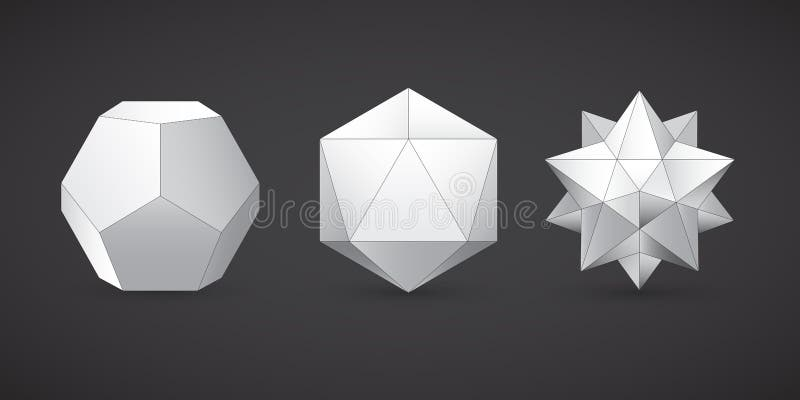Geometric shapes, dodecahedron, vector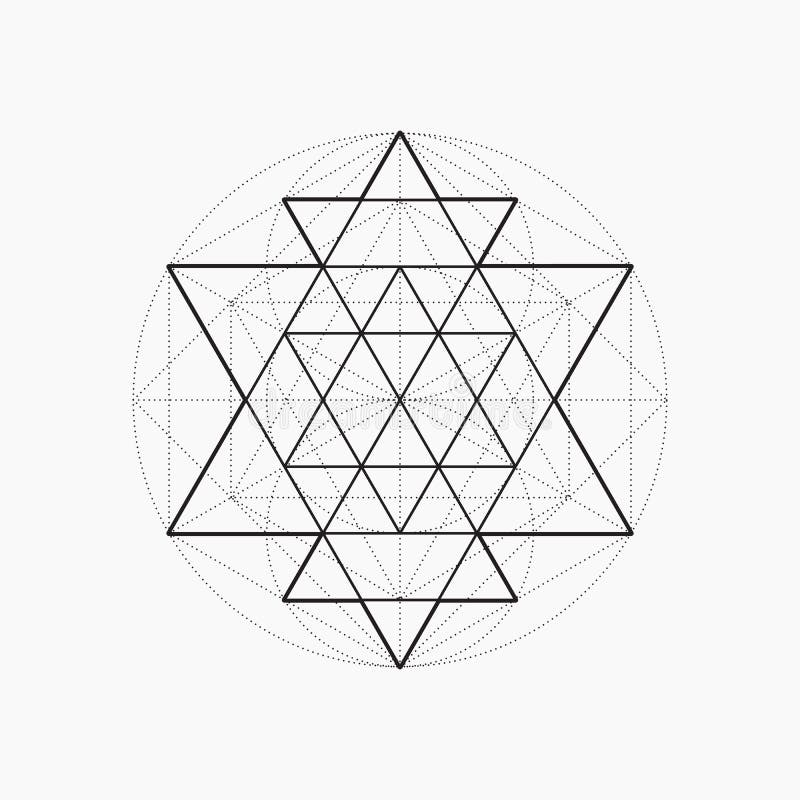Geometric shapes, line design, triangle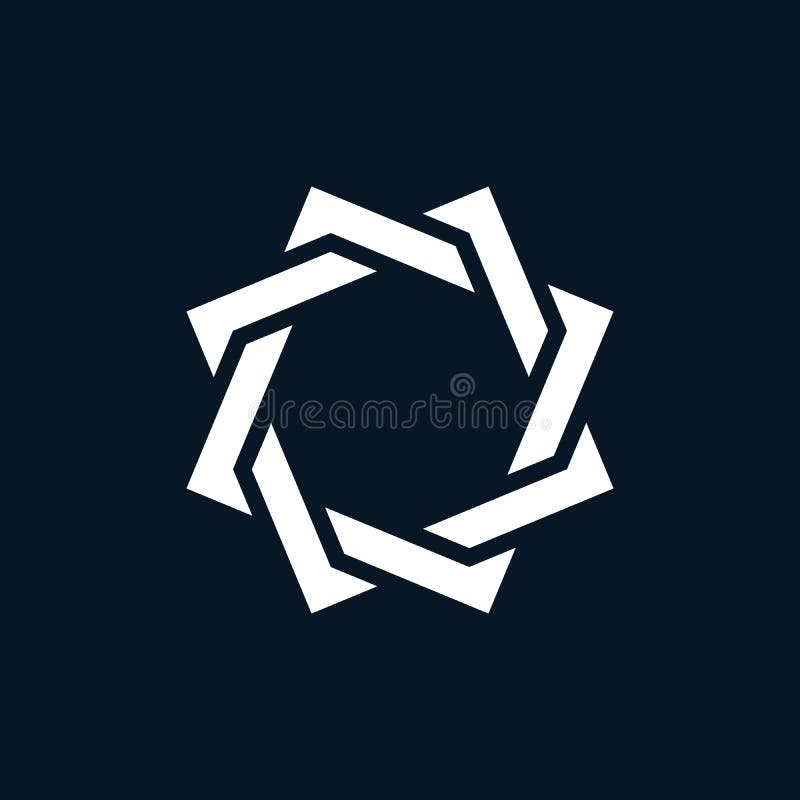Intertwined geometric shapes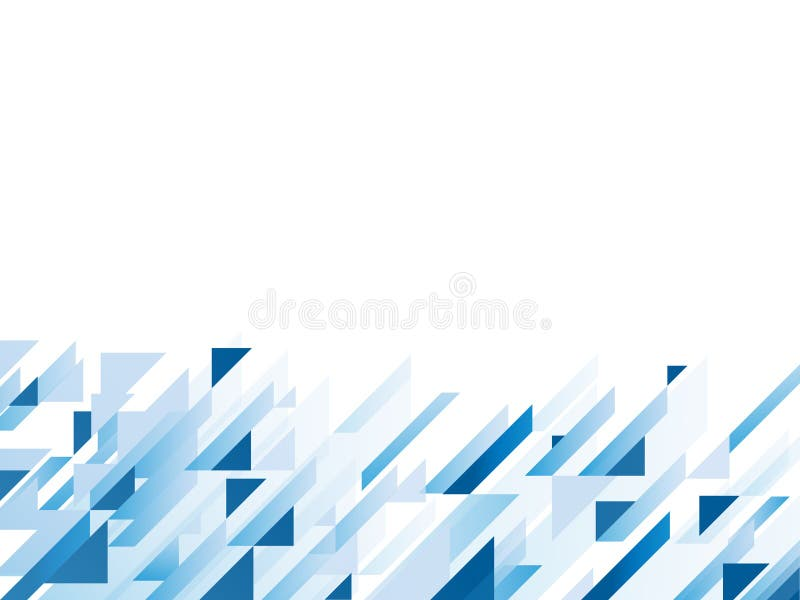Abstract background with blue geometric shapes,